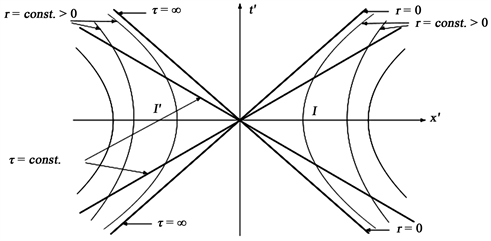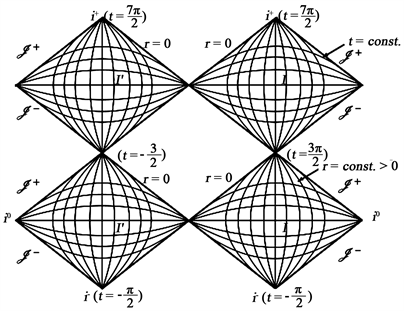﻿ 一类真空Einstein场方程时间周期解的性质

# 一类真空Einstein场方程时间周期解的性质The Properties for a Kind of Time-Periodic Solutions of the Einstein’s Field Equations

Abstract: In general relativity theory, the solution can provide rational explanation for the final state of gravitational collapse if it with physical singularity. In this paper, we study a kind of vacuum Ein-stein field equations time-periodic solution and its physical properties. We computed the Riemann curvature tensor and its length. We proved it’s a time-periodic solution with physical singularity which describes a time-period universe. Through analyzing the Penrose figure of this kind of solutions, we can be found this kind of solutions have similar Physical characters. Because of the time-periodic solutions with physical singularity can provide rational explanation for the final state of gravitational collapse, this space-time can apply to modern cosmology and general relativity.

1. 引言

2. 时间周期解

${G}_{\mu \nu }\triangleq {R}_{\mu \nu }-\frac{1}{2}{\text{g}}_{\mu \nu }R=0$ (1)

${R}_{\mu \nu }=0$ (2)

$\left(t,r,\theta ,\varphi \right)$ 作为求坐标系， $t\in \Re ,r\in \left[0,+\infty \right),\theta \in \left[0,2\text{π}\right),\varphi \in \left[-\text{π}/2,\text{π}/2\right]$ 考虑如下度量形式：

$\text{d}{s}^{2}={u}^{2}\text{d}{t}^{2}+2q\text{d}t\text{d}r+2v\text{d}t\text{d}\varphi -{a}^{2}{b}^{2}\text{d}{r}^{2}-{a}^{2}\text{d}{\theta }^{2}$

$\text{d}{s}^{2}=\left(\text{d}t,\text{d}r,\text{d}\theta ,\text{d}\varphi \right)\left({g}_{\mu \nu }\right){\left(\text{d}t,\text{d}r,\text{d}\theta ,\text{d}\varphi \right)}^{\text{T}}$ (3)

$\left({g}_{\mu \nu }\right)=\left(\begin{array}{cccc}4H{r}^{\frac{3}{2}}+{H}_{0}r\mathrm{ln}r+{H}_{1}r& q& 0& cr\\ q& -\frac{1}{{f}^{6}\sqrt{r}}& 0& 0\\ 0& 0& -\frac{{f}^{2}}{\sqrt{r}}& 0\\ cr& 0& 0& 0\end{array}\right)$ (4)

${H}_{0},{H}_{1},c,q$$f$ 是依赖于t的函数， $H=\frac{24c{f}_{t}^{2}+4{c}_{t}f{f}_{t}-4cf{f}_{tt}}{{f}^{8}c}$

$\text{d}{s}^{2}=\left(\text{d}t,\text{d}r,\text{d}\theta ,\text{d}\varphi \right)\left({\xi }_{\mu \nu }\right){\left(\text{d}t,\text{d}r,\text{d}\theta ,\text{d}\varphi \right)}^{\text{T}}$ (5)

$\left\{\begin{array}{l}{\xi }_{00}=\frac{16{r}^{\frac{3}{2}}\left(6c{f}_{t}^{2}+f{c}_{t}{f}_{t}-cf{f}_{tt}\right)}{{f}^{8}c}+{H}_{0}r\mathrm{ln}r+{H}_{1}r,\\ {\xi }_{01}=q,\\ {\xi }_{03}=cr,\\ {\xi }_{11}=-\frac{1}{{f}^{6}\sqrt{r}},\\ {\xi }_{22}=-\frac{{f}^{2}}{\sqrt{r}},\\ {\xi }_{01}={\xi }_{02}={\xi }_{12}={\xi }_{13}={\xi }_{23}={\xi }_{33}=0\end{array}$ (6)

$\xi \triangleq \mathrm{det}\left({\xi }_{\mu \nu }\right)=-\frac{{c}^{2}r}{{f}^{4}}$ (7)

${\xi }_{00}=\frac{16{r}^{\frac{3}{2}}\left(6c{f}_{t}^{2}+f{c}_{t}{f}_{t}-cf{f}_{tt}\right)}{{f}^{8}c}+{H}_{0}r\mathrm{ln}r+{H}_{1}r>0,$

$|\begin{array}{cc}{\xi }_{00}& {\xi }_{01}\\ {\xi }_{01}& {\xi }_{11}\end{array}|=-\frac{16r\left(6c{f}_{t}^{2}+f{c}_{t}{f}_{t}-cf{f}_{tt}\right)}{{f}^{14}c}-\frac{{H}_{0}\sqrt{r}\mathrm{ln}r+{H}_{1}\sqrt{r}}{{f}^{6}}<0,$

$|\begin{array}{ccc}{\xi }_{00}& {\xi }_{01}& {\xi }_{02}\\ {\xi }_{01}& {\xi }_{11}& {\xi }_{12}\\ {\xi }_{20}& {\xi }_{21}& {\xi }_{22}\end{array}|=\frac{16\sqrt{r}\left(6c{f}_{t}^{2}+f{c}_{t}{f}_{t}-cf{f}_{tt}\right)}{{f}^{12}c}+\frac{{H}_{0}\mathrm{ln}r+{H}_{1}}{{f}^{4}}+\frac{{q}^{2}{f}^{2}}{\sqrt{r}}>0$

$|\begin{array}{cccc}{\xi }_{00}& {\xi }_{01}& {\xi }_{02}& {\xi }_{03}\\ {\xi }_{10}& {\xi }_{11}& {\xi }_{12}& {\xi }_{13}\\ {\xi }_{20}& {\xi }_{21}& {\xi }_{22}& {\xi }_{23}\\ {\xi }_{30}& {\xi }_{31}& {\xi }_{32}& {\xi }_{33}\end{array}|=-\frac{{c}_{2}r}{{f}^{4}}<0,$

3. 奇性分析

${R}_{0101}=\frac{6c{f}_{t}^{2}+f{c}_{t}{f}_{t}-cf{f}_{tt}}{{f}^{8}c\sqrt{r}}+\frac{2{H}_{0}\sqrt{r}\mathrm{ln}r+2{q}^{2}{f}^{6}-{H}_{1}\sqrt{r}}{8{r}^{\frac{3}{2}}}$ (8)

${R}_{0130}=\frac{cq{f}^{6}}{4\sqrt{r}}$ (9)

${R}_{0202}=\frac{-{H}_{1}{f}^{8}c+24\sqrt{r}cf{f}_{tt}-144\sqrt{r}c{f}_{t}^{2}-24\sqrt{r}f{c}_{t}{f}_{t}}{8cr}$ (10)

${R}_{0221}=-\frac{q{f}^{8}}{8{r}^{2}}$ (11)

${R}_{0223}=-\frac{c{f}^{8}}{8r}$ (12)

${R}_{0303}=-\frac{{c}^{2}{f}^{6}\sqrt{r}}{4}$ (13)

${R}_{1212}=\frac{{f}^{2}}{4{r}^{\frac{5}{2}}}$ (14)

$R=\frac{3{f}^{12}}{4{r}^{3}}$ (15)

$R\to +\infty$ (16)

4. 一类特殊时间周期解的物理性质

$\text{d}{s}^{2}=\frac{16{r}^{\frac{3}{2}}}{{\left(1+\mathrm{sin}t\right)}^{7}}\text{d}{t}^{2}+\frac{2r}{{\left(1+\mathrm{sin}t\right)}^{5}}\text{d}t\text{d}\varphi -\frac{1}{\sqrt{r}{\left(1+\mathrm{sin}t\right)}^{6}}\text{d}{r}^{2}-\frac{{\left(1+\mathrm{sin}t\right)}^{2}}{\sqrt{r}}\text{d}{\theta }^{2}.$ (17)

$\Re \triangleq {R}^{\alpha \beta \gamma \delta }{R}_{\alpha \beta \gamma \delta }=\frac{3{\left(1+\mathrm{sin}t\right)}^{12}}{4{r}^{3}},$ (18)

$\theta$$\varphi$ 取定值，可得度规

$\text{d}{s}^{2}=\frac{16{r}^{\frac{3}{2}}}{{\left(1+\mathrm{sin}t\right)}^{7}}\text{d}{t}^{2}-\frac{1}{\sqrt{r}{\left(1+\mathrm{sin}t\right)}^{6}}\text{d}{r}^{2}.$

$\frac{16{r}^{\frac{3}{2}}}{{\left(1+\mathrm{sin}t\right)}^{7}}\text{d}{t}^{2}-\frac{1}{\sqrt{r}{\left(1+\mathrm{sin}t\right)}^{6}}\text{d}{r}^{2}=0.$

$\frac{\text{d}t}{\text{d}r}=±\frac{\sqrt{1+\mathrm{sin}t}}{4r}.$

$\frac{\text{d}r}{4r}=\frac{\text{d}t}{\sqrt{1+\mathrm{sin}t}}$ (10)

$\frac{\frac{\text{d}R\left(t\right)}{\text{d}t}}{R\left(t\right)}=\frac{4}{\sqrt{1+\mathrm{sin}t}}.$ (20)

$\tau =t+\text{π}/2$ ，可得

$\frac{\frac{\text{d}R\left(t\right)}{\text{d}t}}{R\left(t\right)}\approx \frac{4\sqrt{2}}{\tau },\text{\hspace{0.17em}}\forall |\tau |\ll 1.$ (21)

$t\in \Re$ 取为定值，t-截面的诱导度规由(5)可得：

$\text{d}{s}^{2}=-\frac{1}{\sqrt{r}{\left(1+\mathrm{sin}t\right)}^{6}}\left[\text{d}{r}^{2}+{\left(1+\mathrm{sin}t\right)}^{8}\text{d}{\theta }^{2}\right].$ (22)

$\tau ={\int }_{\frac{\text{π}}{2}}^{t}\frac{1}{1+\mathrm{sin}\xi }\text{d}\xi ,$ (23)

$\tau =\sqrt{2}{\int }_{\frac{\text{π}}{2}}^{t}\frac{1}{\mathrm{cos}\left(\frac{\xi }{2}-\frac{\text{π}}{4}\right)}\text{d}\frac{\xi }{2}={\sqrt{2}\mathrm{ln}|\frac{1+\mathrm{sin}\left(\frac{t}{2}-\frac{\text{π}}{4}\right)}{\mathrm{cos}\left(\frac{t}{2}-\frac{\text{π}}{4}\right)}||}_{\frac{\text{π}}{2}}^{t}=\sqrt{2}\mathrm{ln}|\frac{1+\mathrm{sin}\left(\frac{t}{2}-\frac{\text{π}}{4}\right)}{\mathrm{cos}\left(\frac{t}{2}-\frac{\text{π}}{4}\right)}|$

$\tau =\left\{\begin{array}{l}-\infty ,\text{\hspace{0.17em}}\text{\hspace{0.17em}}\text{\hspace{0.17em}}t=-\text{π}/2,\\ 0,\text{\hspace{0.17em}}\text{\hspace{0.17em}}\text{\hspace{0.17em}}\text{\hspace{0.17em}}\text{\hspace{0.17em}}\text{\hspace{0.17em}}t=\text{π}/2,\\ \infty ,\text{\hspace{0.17em}}\text{\hspace{0.17em}}\text{\hspace{0.17em}}\text{\hspace{0.17em}}\text{\hspace{0.17em}}t=\text{3π}/2,\end{array}$ (24)

$\text{d}{s}^{2}=\frac{16{r}^{\frac{3}{2}}}{{\left(1+\mathrm{sin}t\right)}^{6}}\text{d}{\tau }^{2}+\frac{2r}{{\left(1+\mathrm{sin}t\right)}^{4}}\text{d}\tau \text{d}\varphi -\frac{1}{\sqrt{r}{\left(1+\mathrm{sin}t\right)}^{6}}\text{d}{r}^{2}-\frac{{\left(1+\mathrm{sin}t\right)}^{2}}{\sqrt{r}}\text{d}{\theta }^{2}.$ (25)

${r}^{*}=\int \frac{1}{4r}\text{d}r=\frac{1}{4}\mathrm{ln}r.$

$v=\tau +{r}^{*}=\tau +\frac{1}{4}\mathrm{ln}r.$ (26)

$w=\tau -{r}^{*}=\tau -\frac{1}{4}\mathrm{ln}r.$ (27)

$\text{d}{s}^{2}=\frac{16{r}^{\frac{3}{2}}}{{\left(1+\mathrm{sin}t\right)}^{6}}\left(\text{d}{v}^{2}-\frac{1}{2}\text{d}v\text{d}r\right)+\frac{2r}{{\left(1+\mathrm{sin}t\right)}^{4}}\left(\text{d}v-\frac{1}{4}\text{d}r\right)\text{d}\varphi -\frac{{\left(1+\mathrm{sin}t\right)}^{2}}{\sqrt{r}}\text{d}{\theta }^{2}.$ (28)

$\text{d}{s}^{2}=\frac{16{r}^{\frac{3}{2}}}{{\left(1+\mathrm{sin}t\right)}^{6}}\left(\text{d}{w}^{2}-\frac{1}{2}\text{d}w\text{d}r\right)+\frac{2r}{{\left(1+\mathrm{sin}t\right)}^{4}}\left(\text{d}w-\frac{1}{4}\text{d}r\right)\text{d}\varphi -\frac{{\left(1+\mathrm{sin}t\right)}^{2}}{\sqrt{r}}\text{d}{\theta }^{2}.$ (29)

$\text{d}{s}^{2}=\frac{16{r}^{\frac{3}{2}}}{{\left(1+\mathrm{sin}t\right)}^{6}}\text{d}v\text{d}w+\frac{r}{{\left(1+\mathrm{sin}t\right)}^{4}}\left(\text{d}v+\text{d}w\right)\text{d}\varphi -\frac{{\left(1+\mathrm{sin}t\right)}^{2}}{\sqrt{r}}\text{d}{\theta }^{2},$ (30)

$\frac{1}{2}\left(v+w\right)={\int }_{-\frac{\text{π}}{2}}^{t}\frac{1}{\sqrt{1+\mathrm{sin}\xi }}\text{d}\xi ,\text{\hspace{0.17em}}\text{\hspace{0.17em}}v-w=\frac{1}{2}\int \frac{1}{r}\text{d}r=\frac{1}{2}\mathrm{ln}r.$ (31)

$\text{d}{s}^{2}=\frac{16{r}^{\frac{3}{2}}}{{\left(1+\mathrm{sin}t\right)}^{6}}\frac{\text{d}v}{\text{d}{v}^{\prime }}\frac{\text{d}w}{\text{d}{w}^{\prime }}\text{d}{v}^{\prime }\text{d}{w}^{\prime }+\frac{r}{{\left(1+\mathrm{sin}t\right)}^{4}}\left(\frac{\text{d}v}{\text{d}{v}^{\prime }}\text{d}{v}^{\prime }+\frac{\text{d}w}{\text{d}{w}^{\prime }}\text{d}{w}^{\prime }\right)\text{d}\varphi -\frac{{\left(1+\mathrm{sin}t\right)}^{2}}{\sqrt{r}}\text{d}{\theta }^{2}$ (32)

${t}^{\prime }=\frac{1}{2}\left({v}^{\prime }+{w}^{\prime }\right),\text{\hspace{0.17em}}\text{\hspace{0.17em}}{x}^{\prime }=\left({v}^{\prime }-{w}^{\prime }\right).$ (33)

$\text{d}{s}^{2}={F}^{2}\left({t}^{\prime },{x}^{\prime }\right)\left(\text{d}{{t}^{\prime }}^{2}-\text{d}{{x}^{\prime }}^{2}\right)+G\left({t}^{\prime },{x}^{\prime }\right)\text{d}{t}^{\prime }\text{d}\varphi -H\left({t}^{\prime },{x}^{\prime }\right)\text{d}{x}^{\prime }\text{d}\varphi -{L}^{2}\left({t}^{\prime },{x}^{\prime }\right)\text{d}{\theta }^{2}.$ (34)

${{t}^{\prime }}^{2}-{{x}^{\prime }}^{2}=-r$ (35)

${t}^{\prime }+{x}^{\prime }=-\left({t}^{\prime }-{x}^{\prime }\right)\mathrm{exp}\left(4\tau \right)=-\left({t}^{\prime }-{x}^{\prime }\right)\mathrm{exp}\left(4{\int }_{\frac{\text{π}}{2}}^{t}\frac{1}{\sqrt{1+\mathrm{sin}\xi }}\text{d}\xi \right)$ (36)

${F}^{2}=\frac{4\sqrt{R}}{{\left(1+\mathrm{sin}\right)}^{6}},\text{\hspace{0.17em}}\text{\hspace{0.17em}}G=\frac{{x}^{\prime }}{{\left(1+\mathrm{sin}\right)}^{4}},\text{\hspace{0.17em}}\text{\hspace{0.17em}}H=\frac{{t}^{\prime }}{{\left(1+\mathrm{sin}\right)}^{4}},\text{\hspace{0.17em}}\text{\hspace{0.17em}}{L}^{2}=\frac{{\left(1+\mathrm{sin}\right)}^{2}}{\sqrt{r}}.$ (37)

${v}^{″}=\mathrm{arctan}\left({v}^{\prime }\right),\text{\hspace{0.17em}}\text{\hspace{0.17em}}{w}^{″}=\mathrm{arctan}\left({w}^{\prime }\right),$ (38)

$\text{d}{s}^{2}=-\frac{{\left(1+\mathrm{sin}t\right)}^{2}}{\sqrt{r}}\text{d}{\theta }^{2}$Figure 1. Kruskal diagram, you can see the time period space region I and I prime, and area of $r<0$Figure 2. The Penrose diagram of the LW time period universe

$\text{d}{s}^{2}=\left(1-\frac{2m}{r}\right)\text{d}{v}^{2}-2\text{d}v\text{d}r-{r}^{2}\left(\text{d}{\theta }^{2}+{\mathrm{sin}}^{2}\theta \text{d}{\varphi }^{2}\right),$

${\text{d}}^{\text{*}}{s}^{2}=-{r}^{2}\left(\text{d}{\theta }^{2}+{\mathrm{sin}}^{2}\theta \text{d}{\varphi }^{2}\right).$

5. 结论

 Bicak, J. (2000) Selected Solutions of Einstein’s Field Equations: Their Role in General Relativity and Astrophysics. Einstein’s Field Equations and Their Physical Implications, Lecture Notes in Phys. Springer, Berlin, 1-126.

 Schwarzschild, K. (1916) Über das Gravitationsfeld eines Massenpunktes nach der Einsteins chen theorie. Sitz. Preuss. Akad. Wiss, 189, 688-696.

 Hawking, S. and Ellis, G. (1973) The Large Scale Structure of Space-Time. Cambridge University Press, Cambridge.

 Stephani, H., Kramer, D., MacCallum, M., et al. (2003) Exact Solutions of Einstein’s Field Equations. 2nd Edition. Cambridge Monographs on Mathematical Physics. Cambridge University Press, Cambridge.

 Dafermos, M. (2003) Stability and Instability of the Cauchy Horizon for the Spherically Symmetric Einstein-Max- well-Scalar Field Equations. Annals of Mathematics, 158, 875-928.
https://doi.org/10.4007/annals.2003.158.875

 Kong, D.X. and Liu, K.F. (2010) Time-Periodic Solutions of the Einstein’s Field Equations I: General Framework. Science in China Series A, 5, 66-83.

 Kong, D.X, Liu, K.F. and Shen, M. (2010) Time-Periodic Solutions of the Einstein’s Field Equations II: Geometric Singularities. Science China Mathematics, 53, 1507-1520.
https://doi.org/10.1007/s11425-009-3164-y

 Kong, D.X., Liu, K.F. and Shen, M. (2011) Time-Periodic Solutions of the Einstein’s Field Equations III: Physical Singularities. Science China Mathematics, 54, 23-33.
https://doi.org/10.1007/s11425-010-4003-x

 Wald, R.M. (1984) Gencral Rtlativity. The University of Chicago Press, Chicago and London.
https://doi.org/10.7208/chicago/9780226870373.001.0001

 Wang, Z.G. (2009) Physical Characters of KLS Time-Periodic Universe. Science in China Series A, 39, 1039-1044

Top